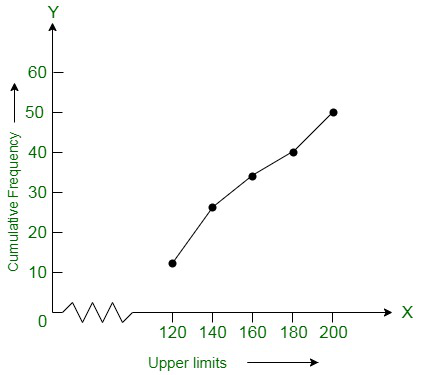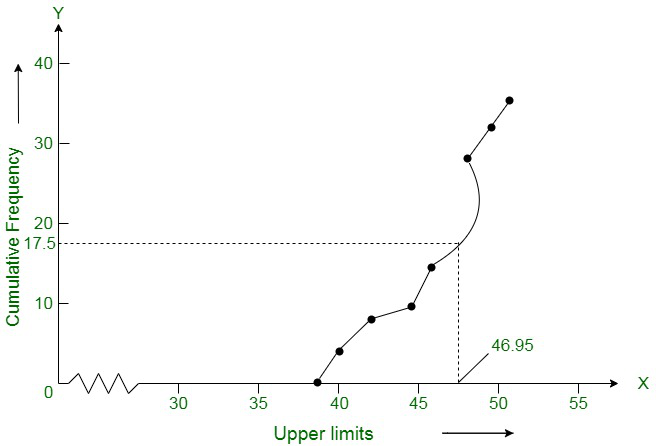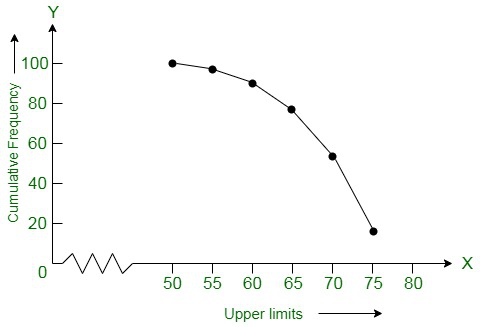Related Articles

# Class 10 NCERT Solutions- Chapter 14 Statistics – Exercise 14.4

• Last Updated : 03 Mar, 2021

### Question 1. The following distribution gives the daily income of 50 workers if a factory. Convert the distribution above to a less than type cumulative frequency distribution and draw its ogive.

Solution:

According to the question, we convert the given distribution to a less than type cumulative frequency distribution,

Attention reader! All those who say programming isn't for kids, just haven't met the right mentors yet. Join the  Demo Class for First Step to Coding Coursespecifically designed for students of class 8 to 12.

The students will get to learn more about the world of programming in these free classes which will definitely help them in making a wise career choice in the future.

Now according to the table we plot the points that are corresponding to the ordered pairs (120, 12), (140, 26), (160, 34), (180, 40), and (200, 50) on a graph paper. Here x-axis represents the upper limit and y-axis represent the frequency. The curve obtained from the graph is known as less than type ogive curve.### Draw a less than type ogive for the given data. Hence obtain the median weight from the graph and verify the result by using the formula.

Solution:

According to the give table, we get the points (38, 0), (40, 3), (42, 5), (44, 9), (46, 14), (48, 28), (50, 32) and (52, 35). Now using these points we draw an ogive, where the x-axis represents the upper limit and y-axis represents the frequency. The curve obtained is known as less than type ogive.Now, locate the point 17.5 on the y-axis and draw a line parallel to the x-axis cutting the curve at a point. From this point, now we draw a perpendicular line to the x-axis and the intersection point which is perpendicular to x-axis is the median of the given data. After, locating point now we create a table to find the mode:

The class 46 – 48 has the maximum frequency, hence, this is the modal class

= 46, h = 2, f1 = 14, f0 = 5 and f2 = 4

Now we find the mode:

Mode =On substituting the values in the given formula, we get

= 46 + 0.95 = 46.95

Hence, the mode is verified.

### Change the distribution to a more than type distribution and draw its ogive.

Solution:

According to the question, we change the distribution to a more than type distribution.

Now, according to the table we draw the ogive by plotting the points. Here, the a-axis represents the upper limit and y-axis represents the cumulative frequency. And the points are(50, 100), (55, 98), (60, 90), (65, 78), (70, 54) and (75, 16) on this graph paper. The graph obtained is known as more than type ogive curve.My Personal Notes arrow_drop_up